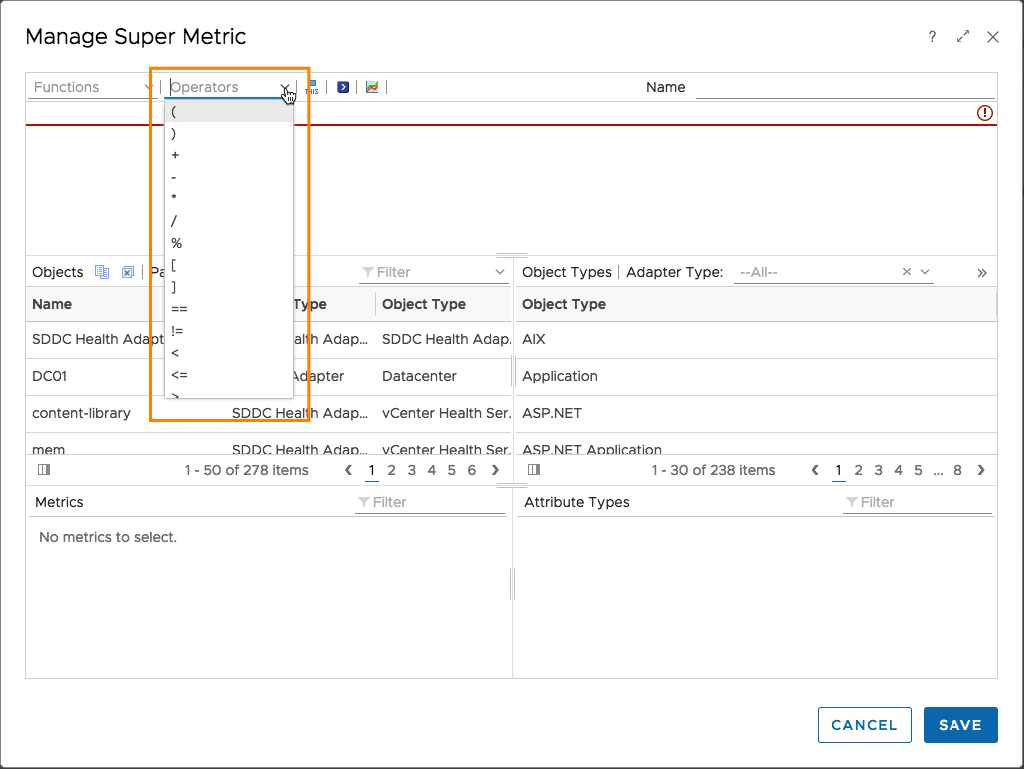# vROps SuperMetric using logical expressions

vRealie Operations Super Metrics are a very flexible and powerful way to extend the capabilities of the product way beyond the OOB content.

There are many blog articles out there explaining how to basically use super metrics but only very few sources gives some examples how to put logical expressions into your formulas. So the question is, how dos this work?Using some simple examples I am going to explain how the magic of logical expressions work in vROps Super Metrics.

###### First of all some fundamentals:
• Super Metric working on a selected object itself, like ESXi cluster in this example, which is just showing the actual metric (we will need soon):
`avg(\${this, metric=summary|total_number_hosts})`
• Super Metric working on direct descendants of a selected object, in this case ESXi hosts in a cluster, which is counting the powered on hosts:
`count(\${adaptertype=VMWARE, objecttype=HostSystem, metric=sys|poweredOn, depth=1})`
###### Now, let’s put the pieces together and build a super metric which follows this pattern:
`condition ? inCaseOfTrue : elseCase;`

Even if this doesn’t mean anything in terms of semantics, the syntax of such an expression might look like this one:

`count(\${adaptertype=VMWARE, objecttype=HostSystem, metric=sys|poweredOn, depth=1}) == avg(\${this, metric=summary|total_number_hosts}) && avg(\${adaptertype=VMWARE, objecttype=HostSystem, metric=cpu|usage_average, depth=1} as cpuUsage) > 40 ? avg(cpuUsage ):5`One could translate this formula into that statement:

`If all ESXi hosts in a given cluster are powered on AND clusters average CPU usage is greater than 40 THEN show me the average CPU usage, ELSE show me 5`